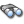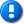alvinalexander.com | career | drupal | java | mac | mysql | perl | scala | uml | unix

# JMeter example source code file (FunctionParser.java)

This example JMeter source code file (FunctionParser.java) is included in the DevDaily.com "Java Source Code Warehouse" project. The intent of this project is to help you "Learn Java by Example" TM.

## The JMeter FunctionParser.java source code

```/*
* Licensed to the Apache Software Foundation (ASF) under one or more
* contributor license agreements.  See the NOTICE file distributed with
* The ASF licenses this file to You under the Apache License, Version 2.0
* (the "License"); you may not use this file except in compliance with
* the License.  You may obtain a copy of the License at
*
*
* Unless required by applicable law or agreed to in writing, software
* WITHOUT WARRANTIES OR CONDITIONS OF ANY KIND, either express or implied.
* See the License for the specific language governing permissions and
*
*/

/*
* Created on Jul 25, 2003
*/
package org.apache.jmeter.engine.util;

import java.io.IOException;

import org.apache.jmeter.engine.StandardJMeterEngine;
import org.apache.jmeter.functions.Function;
import org.apache.jmeter.functions.InvalidVariableException;
import org.apache.jmeter.testelement.TestListener;
import org.apache.jorphan.logging.LoggingManager;
import org.apache.log.Logger;

/**
* Parses function / variable references of the form
* \${functionName[([var[,var...]])]}
* and
* \${variableName}
*/
class FunctionParser {
private static final Logger log = LoggingManager.getLoggerForClass();

/**
* Compile a general string into a list of elements for a CompoundVariable.
*
*
* Removes escapes from '\$', ',' and '\'.
*
* @param value string containing the function / variable references (if any)
*
* @return list of Strings or Objects representing functions
*/
LinkedList<Object> compileString(String value) throws InvalidVariableException {
StringBuilder buffer = new StringBuilder();
char previous = ' '; // TODO - why use space?
char[] current = new char;
try {
if (current == '\\') { // Handle escapes
previous = current;
break;
}
// Keep the '\' unless it is one of the escapable chars '\$' ',' or '\'
// N.B. This method is used to parse function parameters, so must treat ',' as special
if (current != '\$' && current != ',' && current != '\\') {
buffer.append(previous); // i.e. '\\'
}
previous = ' ';
buffer.append(current);
continue;
} else if (current == '{' && previous == '\$') {// found "\${"
buffer.deleteCharAt(buffer.length() - 1);
if (buffer.length() > 0) {// save leading text
buffer.setLength(0);
}
previous = ' ';
} else {
buffer.append(current);
previous = current;
}
}
if (buffer.length() > 0) {
}
} catch (IOException e) {
log.error("Error parsing function: " + value, e);
result.clear();
}
if (result.size() == 0) {
}
return result;
}

/**
* Compile a string into a function or SimpleVariable.
*
* Called by {@link #compileString(String)} when that has detected "\${".
*
* Calls {@link CompoundVariable#getNamedFunction(String)} if it detects:
* '(' - start of parameter list
* '}' - end of function call
*
* @param reader points to input after the "\${"
* @return the function or variable object (or a String)
*/
char[] current = new char;
char previous = ' '; // TODO - why use space?
StringBuilder buffer = new StringBuilder();
Object function;
try {
if (current == '\\') {
break;
}
previous = ' ';
buffer.append(current);
continue;
} else if (current == '(' && previous != ' ') {
String funcName = buffer.toString();
function = CompoundVariable.getNamedFunction(funcName);
if (function instanceof Function) {
reader.reset();// set to start of string
char []cb = new char;
throw new InvalidVariableException
("Expected } after "+funcName+" function call in "+new String(cb));
}
if (function instanceof TestListener) {
StandardJMeterEngine.register((TestListener) function);
}
return function;
} else { // Function does not exist, so treat as per missing variable
buffer.append(current);
}
continue;
} else if (current == '}') {// variable, or function with no parameter list
function = CompoundVariable.getNamedFunction(buffer.toString());
if (function instanceof Function){// ensure that setParameters() is called.
}
buffer.setLength(0);
return function;
} else {
buffer.append(current);
previous = current;
}
}
} catch (IOException e) {
log.error("Error parsing function: " + buffer.toString(), e);
return null;
}
log.warn("Probably an invalid function string: " + buffer.toString());
return buffer.toString();
}

/**
* Compile a String into a list of parameters, each made into a
* CompoundVariable.
*
* Parses strings of the following form:
* <ul>
* <li>text)
* <li>text,text)
* <li>
* </ul>
* @param reader a StringReader pointing to the current input location, just after "("
* @return a list of CompoundVariable elements
*/
StringBuilder buffer = new StringBuilder();
char[] current = new char;
char previous = ' ';
int functionRecursion = 0;
int parenRecursion = 0;
try {
if (current == '\\') { // Process escaped characters
buffer.append(current); // Store the \
break; // end of buffer
}
previous = ' ';
buffer.append(current); // store the following character
continue;
} else if (current == ',' && functionRecursion == 0) {
CompoundVariable param = new CompoundVariable();
param.setParameters(buffer.toString());
buffer.setLength(0);
} else if (current == ')' && functionRecursion == 0 && parenRecursion == 0) {
// Detect functionName() so this does not generate empty string as the parameter
if (buffer.length() == 0 && result.isEmpty()){
return result;
}
// Normal exit occurs here
CompoundVariable param = new CompoundVariable();
param.setParameters(buffer.toString());
buffer.setLength(0);
return result;
} else if (current == '{' && previous == '\$') {
buffer.append(current);
previous = current;
functionRecursion++;
} else if (current == '}' && functionRecursion > 0) {
buffer.append(current);
previous = current;
functionRecursion--;
} else if (current == ')' && functionRecursion == 0 && parenRecursion > 0) {
buffer.append(current);
previous = current;
parenRecursion--;
} else if (current == '(' && functionRecursion == 0) {
buffer.append(current);
previous = current;
parenRecursion++;
} else {
buffer.append(current);
previous = current;
}
}
} catch (IOException e) {// Should not happen with StringReader
log.error("Error parsing function: " + buffer.toString(), e);
}
// Dropped out, i.e. did not find closing ')'
log.warn("Probably an invalid function string: " + buffer.toString());
CompoundVariable var = new CompoundVariable();
var.setParameters(buffer.toString());
return result;
}
}

Other JMeter examples (source code examples)
Here is a short list of links related to this JMeter FunctionParser.java source code file:The search pageOther JMeter source code examples at this package levelClick here to learn more about this project

```
 ... this post is sponsored by my books ...#1 New Release!FP Best Seller